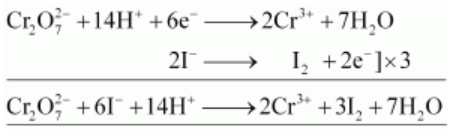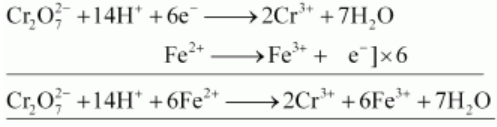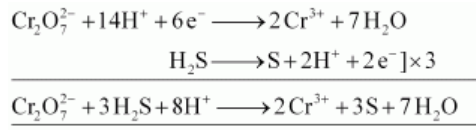# Describe the oxidising action of potassium dichromate and write the ionic equations for its reaction with:Question:

Describe the oxidising action of potassium dichromate and write the ionic equations for its reaction with:

(i) iodide (ii) iron(II) solution and (iii) H2S

Solution:

$\mathrm{K}_{2} \mathrm{Cr}_{2} \mathrm{O}_{7}$ acts as a very strong oxidising agent in the acidic medium.

$\mathrm{K}_{2} \mathrm{Cr}_{2} \mathrm{O}_{7}+4 \mathrm{H}_{2} \mathrm{SO}_{4} \longrightarrow \mathrm{K}_{2} \mathrm{SO}_{4}+\mathrm{Cr}_{2}\left(\mathrm{SO}_{4}\right)_{3}+4 \mathrm{H}_{2} \mathrm{O}+3[\mathrm{O}]$

$\mathrm{K}_{2} \mathrm{Cr}_{2} \mathrm{O}_{7}$ takes up electrons to get reduced and acts as an oxidising agent. The reaction of $\mathrm{K}_{2} \mathrm{Cr}_{2} \mathrm{O}_{7}$ with other iodide, iron (II) solution, and $\mathrm{H}_{2} \mathrm{~S}$ are given below.

(i) $\mathrm{K}_{2} \mathrm{Cr}_{2} \mathrm{O}_{7}$ oxidizes iodide to iodine.(ii) $\mathrm{K}_{2} \mathrm{Cr}_{2} \mathrm{O}_{7}$ oxidizes iron (II) solution to iron (III) solution i.e., ferrous ions to ferric ions.(iii) $\mathrm{K}_{2} \mathrm{Cr}_{2} \mathrm{O}_{7}$ oxidizes $\mathrm{H}_{2} \mathrm{~S}$ to sulphur.